Main Body

# Percent Impedance

The percent impedance (%Z) is the percent of the rated load impedance possessed by a transformer. The percent impedance is important in that it allows us to:

1. Calculate available fault currents (both individual and bank).
2. Determine whether two transformers are suitable for paralleling.

In the last chapter, we learned how to determine the short-circuit voltage of a transformer. We now can use that voltage to determine the percent impedance:

Eshort circuit/ERated = %Z

## Fault-Current Calculations

To calculate the fault current available from a transformer if a dead short occurs across the secondary terminals, use the formula:

Irated (Secondary)/%Z = Fault current

Remember to use the percentage as a decimal not the full number. For example, 2.5% is actually .025.

How to calculate fault current using %Z:

## Example #1

What is the available fault current of a step-down transformer rated at 50 kVA, 1200 V – 120 V and 2.75% percent impedance?

I(full load) =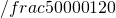I(short circuit) =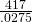I(short circuit) = 15,151 Amps

## Exampe #2

A transformer is rated 20KVA, 4800 V – 240 V.  With the secondary short-circuited, it takes 96 volts to reach the rated primary current (4.2 amps).  Determine the %Z and the available fault current.

%Z =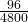%Z = 2

I(rated secondary) =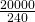I(rated secondary) = 83 Amps

I(Short circuit) =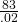I(Short circuit) = 4150 Amps